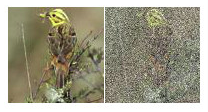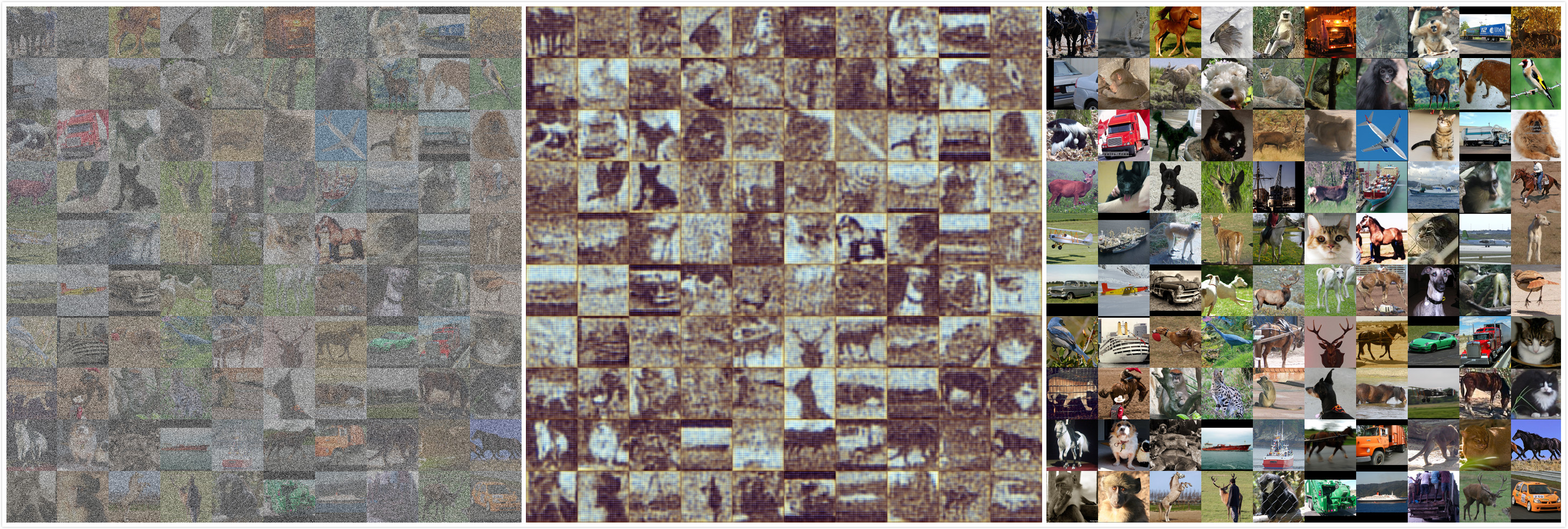【转载请注明出处】chenrudan.github.io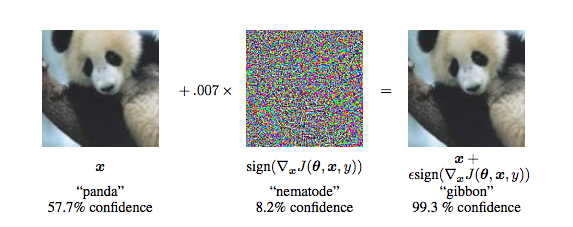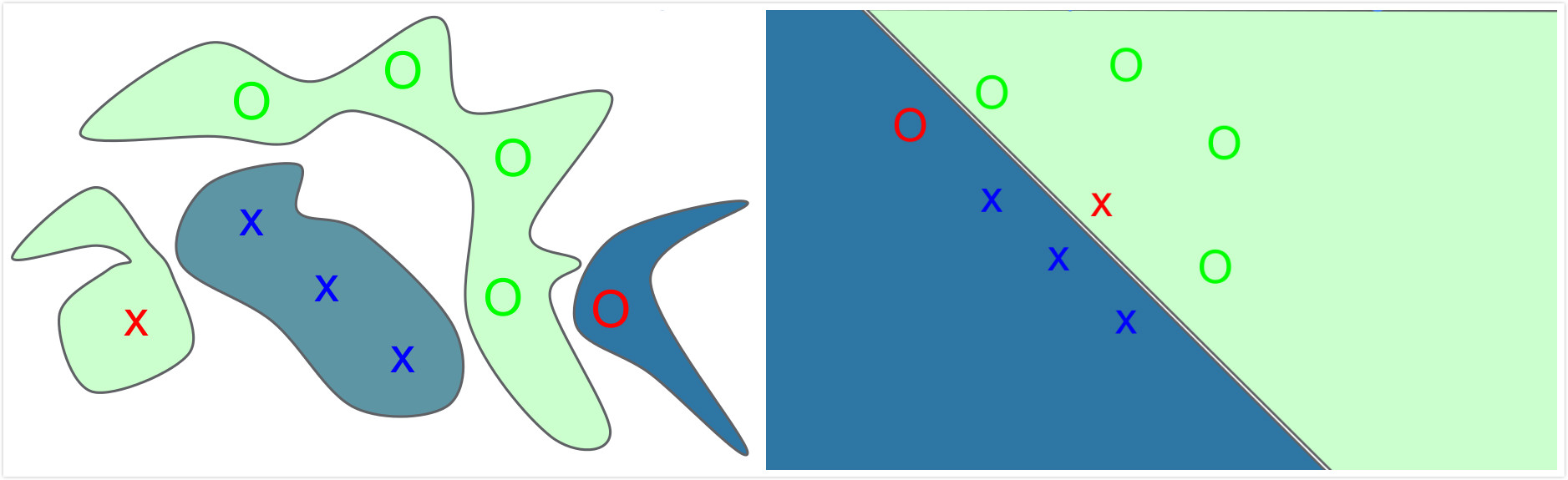$$\eta = \epsilon sign(\triangledown_{x}J(\theta,x,y)) :::::(1)$$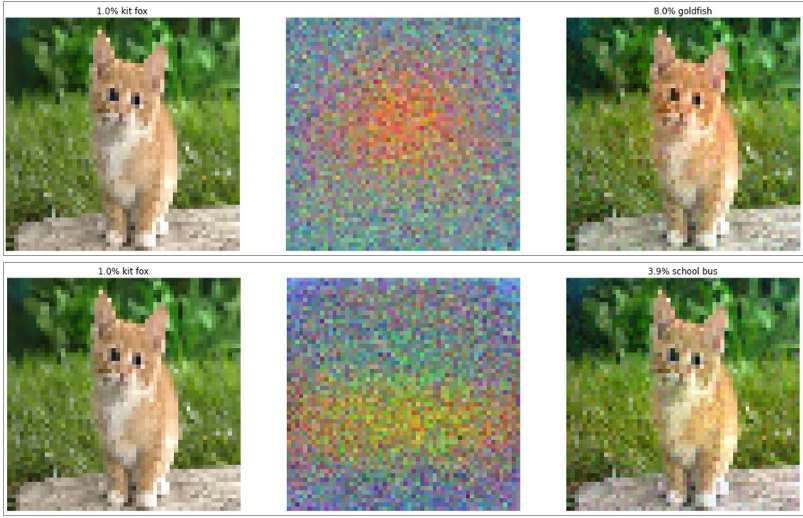$$\widetilde{J}(\theta,x,y) = \alpha J(\theta,x,y)+(1-\alpha)J(\theta,x+\epsilon sign(\triangledown_{x}J(\theta,x,y))) :::::(2)$$

### 2. 生成式对抗网络GAN

14年Goodfellow提出Generative adversarial nets即生成式对抗网络，它要解决的问题是如何从训练样本中学习出新样本，训练样本是图片就生成新图片，训练样本是文章就输出新文章等等。如果能够知道训练样本的分布$p(x)$，那么就可以在分布中随机采样得到新样本，大部分的生成式模型都采用这种思路，GAN则是在学习从随机变量$z$到训练样本$x$的映射关系，其中随机变量可以选择服从正太分布，那么就能得到一个由多层感知机组成的生成网络$G(z;\theta_g)$，网络的输入是一个一维的随机变量，输出是一张图片。如何让输出的伪造图片看起来像训练样本，Goodfellow采用了这样一种方法，在生成网络后面接上一个多层感知机组成的判别网络$D(x;\theta_d)$，这个网络的输入是随机选择一张真实样本或者生成网络的输出，输出是输入图片来自于真实样本$p_{data}$或者生成网络$p_g$的概率，当判别网络能够很好的分辨出输入是不是真实样本时，也能通过梯度的方式说明什么样的输入更加像真实样本，从而通过这个信息来调整生成网络。从而$G$需要尽可能的让自己的输出像真实样本，而$D$则尽可能的将不是真实样本的情况分辨出来。下图左边是GAN算法的概率解释，右边是模型构成。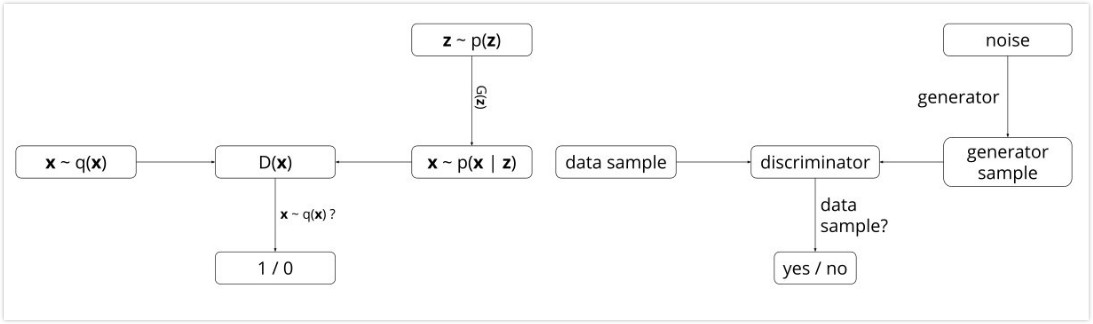GAN的优化是一个极小极大博弈问题，最终的目的是generator的输出给discriminator时很难判断是真实or伪造的，即极大化$D$的判断能力，极小化将$G$的输出判断为伪造的概率，公式如下。论文中将下面式子转化成了Jensen-shannon散度的形式证明了仅当$p_g=p_{data}$时能得到全局最小值，即生成网络能完全的还原出真实样本分布，并且证明了下式能够收敛。(算法流程论文讲的很清楚，这里就不说了，后面结合代码一起解释。)

$$\underset{G}{min} : \underset{D}{max}V(D,G) =E_{x\sim p_{data}(x)}[logD(x)]+E_{z\sim p_{z}(z)}[log(1-D(G(z)))] :::::(3)$$

### 3. 代码解释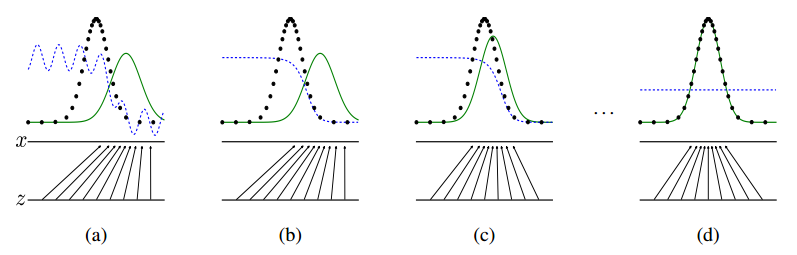a.起始情况

$D$是一个卷积神经网络，变量名是D，其中一层构造方式如下。

$G$是一个逆卷积神经网络，变量名是G，其中一层构造方式如下。

$G$的网络输入为一个$z_{dim}$维服从-1~1均匀分布的随机变量，这里取的是100.

$D$的网络输入是一个batch的64*64的图片，既可以是手写体数据也可以是$G$的一个batch的输出。

b.训练判别网络

c.训练生成网络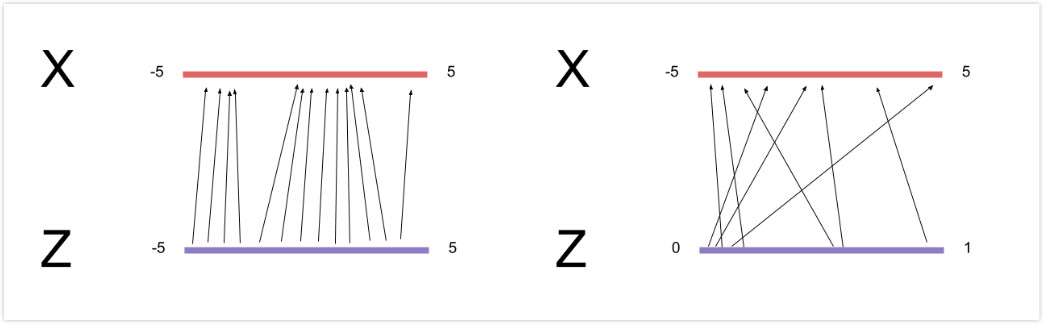### 4. 运行实例